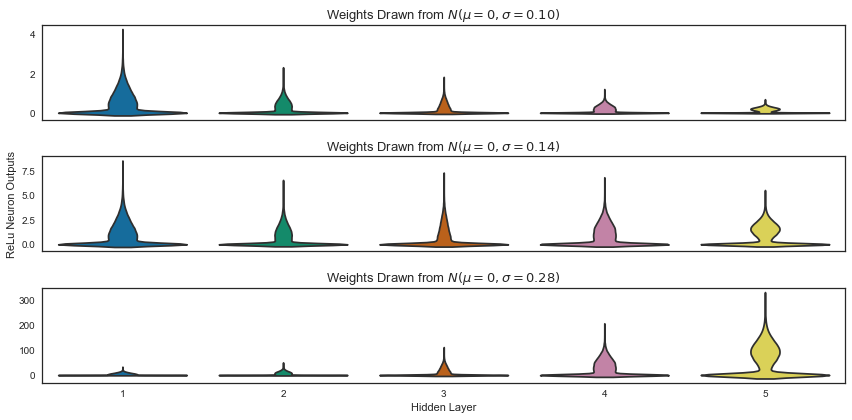# 神经网络中的“垂死的ReLU”问题是什么？

118

“不幸的是，ReLU单元在训练过程中可能很脆弱，并且可能“死亡”。例如，流过ReLU神经元的大梯度可能导致权重更新，从而使神经元再也不会在任何数据点上激活。如果发生这种情况，那么从该点开始流过该单元的梯度将永远为零，也就是说，ReLU单元在训练过程中可能会不可逆地死亡，因为它们可能会从数据流形上脱落下来。例如，您可能会发现多达40个如果学习率设置得太高，您的网络中的％可能是“死亡”的（即永远不会在整个训练数据集中激活的神经元）。通过适当设置学习率，这通常不会成为问题。

2

MarekŽidek'17

3
Dudes我已经设法通过在每个时期为权重<= 0提供新的随机（正态分布）值来使死去的relu神经元恢复活力。随着训练持续到更高的时期，我仅将此方法与冻结不同深度的权重一起使用（I'我不确定这是否就是我们所说的相变。这真的很容易实现。

1
@boli，您可以在这里分享您的实现吗？

120

7

14
@alex：因为偏差对于准确性非常重要。消除偏差与说所有决策平面都必须通过原点几乎相同-除了少数例外，这是一个错误的选择。实际上，摆脱神经网络或相关模型（如线性回归或逻辑回归）中的偏差项通常将意味着您的模型将遭受偏差！这是最终导致同时欠拟合和过度拟合的模型的几种方法之一，，

1
@Alex：我认为向ReLU添加一个小的正偏差是很常见的。我不知道这是否对“垂死的ReLU问题”有所帮助-它可能不会在数值上很大地改变梯度值（因为ReLU的梯度是1或0，并且当它是1时它可能会过冲，较小的起始偏差似乎几乎没有什么不同）。大多数情况下，我认为这只是对初始学习进行少量改进的一种技巧-但这可能会有所帮助，因为它有一个更好的开始，并且通常会更快地降低梯度。

1
@max：您缺少我回答的“任何输入”部分。没有梯度会流到前馈网络中与“死”神经元相关的任何权重，因为到这些权重的所有路径都被切掉-梯度没有其他路径流向向ReLU单元提供权重的子集。您可能会在例如CNN中查看ReLU或将其视为共享权重，在这种情况下，特征图中的所有位置都需要立即设为零。但是，我认为这是“对于任何输入”的另一个实例。

1
@anu：通过梯度下降。由较大的误差值引起的较大的正梯度会依次导致偏差项的单个步骤足够大，以至于“杀死”神经元，从而使其达到将来输入的状态（权重和偏差）到RELU功能向来都在0

102

${z}_{n}=\sum _{i=0}^{k}{w}_{i}{a}_{i}^{n}$
${w}_{i}$$w_i$${a}_{i}^{n}$$a^n_i$${x}_{n}$$x_n$$ReLU=max\left(0,{z}_{n}\right)$$ReLU = max(0,z_n)$

$error=ReLU-y$

$\frac{\mathrm{\partial }error}{\mathrm{\partial }{z}_{n}}={\delta }_{n}=\left\{\begin{array}{cl}1& {z}_{n}\ge 0\\ 0& {z}_{n}<0\end{array}$
${w}_{j}$$w_j$
$\mathrm{\nabla }error=\frac{\mathrm{\partial }error}{\mathrm{\partial }{w}_{j}}=\frac{\mathrm{\partial }error}{\mathrm{\partial }{z}_{n}}×\frac{\mathrm{\partial }{z}_{n}}{\mathrm{\partial }{w}_{j}}={\delta }_{n}×{a}_{j}^{n}=\left\{\begin{array}{cc}{a}_{j}^{n}& {z}_{n}\ge 0\\ 0& {z}_{n}<0\end{array}$

ReLU可能还活着，然后由于某些输入批次的梯度步骤而死亡，从而将权重驱动为较小的值，从而使所有输入的。学习率高会放大此问题。${z}_{n}<0$$z_n < 0$

MohamedEzz 18-3-20

@MohamedEzz，我不明白您的观点What if, for the input 𝑥𝑛, the current weights put the ReLU on the left flat side while it optimally should be on the right side for this particular input ?，如果输入为负，则梯度将为0？在这种情况下最适合什么？您能帮助您理解吗？

1

MohamedEzz

David Refaeli

13

ReLU神经元输出零，并且所有负输入的零导数都为零。因此，如果网络中的权重始终导致ReLU神经元的负输入，则该神经元实际上无助于网络的训练。在数学上，来自该神经元的权重更新对梯度的贡献始终为零（有关详细信息，请参见数学附录）。# 数学附录

$\frac{\mathrm{\partial }L}{\mathrm{\partial }{w}_{jk}^{\left(i\right)}}=\frac{\mathrm{\partial }L}{\mathrm{\partial }{x}_{k}^{\left(i+1\right)}}\frac{\mathrm{\partial }{x}_{k}^{\left(i+1\right)}}{\mathrm{\partial }{w}_{jk}^{\left(i\right)}}\phantom{\rule{thinmathspace}{0ex}}.$

$\begin{array}{rl}\frac{\mathrm{\partial }{x}_{k}^{\left(i+1\right)}}{\mathrm{\partial }{w}_{jk}^{\left(i\right)}}& =\frac{\mathrm{\partial }f\left({s}_{j}^{\left(i\right)}\right)}{\mathrm{\partial }{s}_{j}^{\left(i\right)}}\frac{\mathrm{\partial }{s}_{j}^{\left(i\right)}}{\mathrm{\partial }{w}_{jk}^{\left(i\right)}}\\ & ={f}^{\prime }\left({s}_{j}^{\left(i\right)}\right)\phantom{\rule{thinmathspace}{0ex}}{x}_{j}^{\left(i\right)}.\end{array}$

@anu权重更新为，因此，如果您迈出较大的一步，则在这种情况下，选择一个大的，并且如果是正数，则可以看到可能变为负。如果我们将偏差更新为较大的负值，则尤其不利。$w-\lambda \cdot \mathrm{d}w$$w - \lambda \cdot \mathrm dw$$\lambda$$\lambda$$\mathrm{d}w$$\mathrm dw$$w$$w$

@JohnsonJia，太好了，我明白了:)，还需要澄清一下，为什么在偏重情况下与体重相比特别糟糕，因为对体重和偏见都有负面影响，如果我错了，请纠正我。

5

4

“ Ding ReLU”是指在训练集中为您的数据输出0的神经元。发生这种情况是因为对于所有输入模式，神经元中输入权重 *的总和（也称为激活）变为<= 0。这导致ReLU输出0。在这种情况下，由于ReLU的导数为0，因此不会进行权重更新，并且神经元会停留在输出0处。

1. 垂死的ReLU并不意味着神经元的输出在测试时也将保持零。根据分布差异，情况可能会也可能并非如此。
2. 垂死的ReLU并非永久死亡。如果您添加新的训练数据或使用预先训练的模型进行新的训练，这些神经元可能会反冲！
3. 从技术上讲，即将死亡的ReLU不必为所有训练数据输出0。对于某些数据，它确实可能会输出非零值，但时期数不足以显着移动权重。